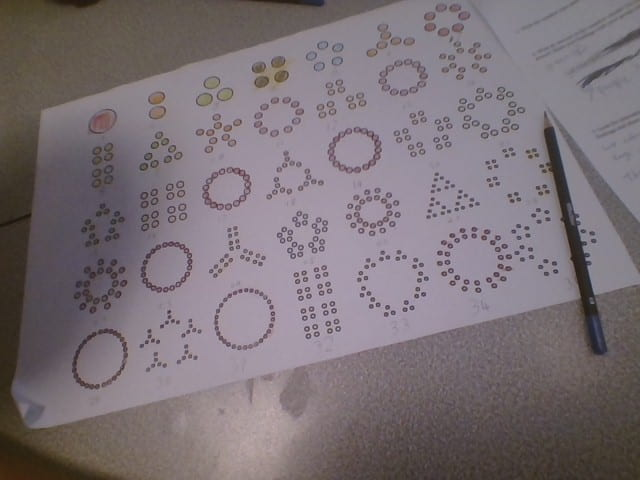# Tag: Prime numbers

## Multiples and Factors

Today we learnt about multiples, factors and prime numbers. A prime number can only be divided by itself and 1. A factor is a number that divides into another number like 2 is a factor of 4. A multiple is a number that can add by using times, for example 2 is a factor of 6 or 3 is a factor of 6.

## Number VisualsHere are our number visuals. We coloured the multiplies of 3,4,2 and more different types of colours, for example we coloured the number 2 orange because we saw a range of numbers that related to it by multiplying. We had to find a pattern in these shapes and numbers we also had to pair into different groups so we could work together with each other.